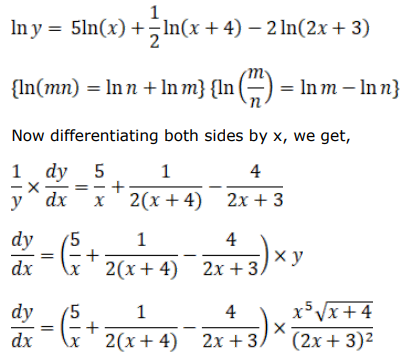# Solve this followingQuestion:

Find $\frac{d y}{d x}$, when:

$y=\frac{x^{5} \sqrt{x+4}}{(2 x+3)^{2}}$

Solution:

Here, we need to take log both the sides to get that differentiation simple.